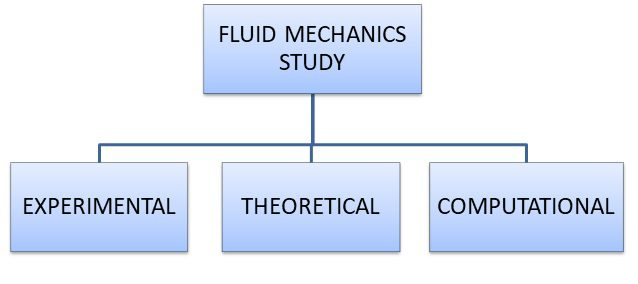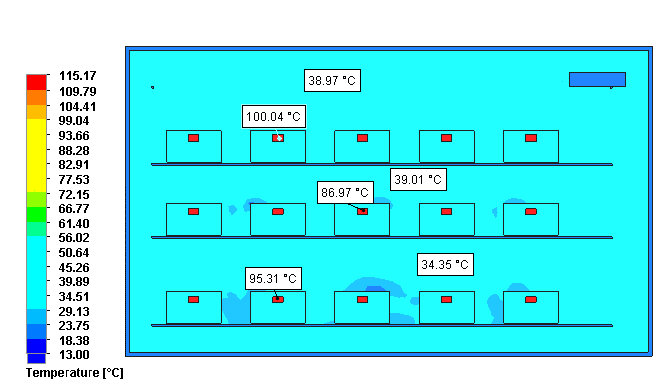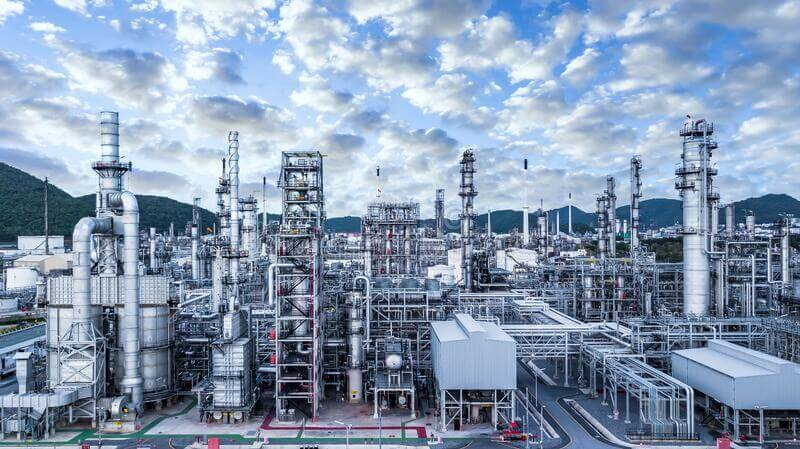For any query, email us at info@wireconsultants.com/ or call us on

### Computational Fluid Dynamics

Fluid mechanics is the science of fluid flow, heat transfer and mass transfer & transport phenomenon. Study of fluid mechanics to obtain temperature, velocity & corresponding profiles is carried out with three different approaches.

Where computational fluid technique have advantage of zero error due to surrounding factors & devices like in experimental method and no assumption for converting partial differential equation into ordinary differential equation like theoretical method. Computation fluid dynamics predict the fluid flow related phenomenon by solving the partial differential equations of fluid properties on a complex computational platform like computer.

Computational fluid dynamics extend the calculation scope from fluid dynamics to heat & mass transfer including the dimension of multi physics & chemical reaction.Computational fluid dynamics aka CFD study is conducted over fluids and fluid flow systems such as conduits, openings, closed walls etc. to calculate fluid flow parameters like velocity, pressure, temperature & viscosity. It gives results to simulate flow patterns and analyze its effects on complete system which help in re-creating and testing the effects of a design before having to actually physically build it. A CFD analysis would help to select an optimum design in Chemical and process engineering, automobile industry, Aerodynamic engineering, HVAC system designing & locomotive design industries.

Enquiry Now• Enquiry Now